Sunday, 29 November 2009

Quantum Atom Theory the symmetry of time and the geometry of spacetime

~

People say that time cannot have geometry and symmetry because time is only one dimensional.

But in Quantum Atom Theory time is only one dimensional for each individual observer.

Each observer has created their own spacetime geometry this can be seen as line symmetry in the physical shape of the observer.

They will see and feel this line symmetry as the arrow of time or as the time line pointing from the past into the future.

There has to be a general principle underlying the formation of such patterns of broken symmetry.

It cannot be just because mathematics works that way that we have the beauty of such symmetry there has to be a physical reason.

The symmetry of mathematics is a reflection of our Universe the Universe is not a reflection of our mathematics.Light sphere of EMR
~

In Quantum Atom Theory it is the emission and absorption of light or EMR from one atom to another that forms the forward momentum of time continuously creating the broken symmetry of spacetime.

Light moves in straight lines always taking the simplest & most efficient path but in three dimensional space it will expand out in all directions as light spheres.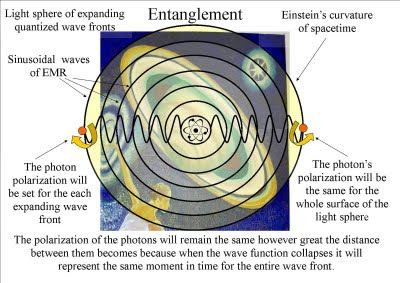Quantum entanglement.
~

The polarization of the light will be the same for the entire surface of the light sphere creating quantum entanglement and the geometry and symmetry of spacetime.Electromagnetic radiation
~

The frequency and wavelength will form spherical waves of periodic motion. Because of the wave nature of EMR it is impossible to achieve absolute zero therefore all systems will oscillate in periodic or harmonic motion.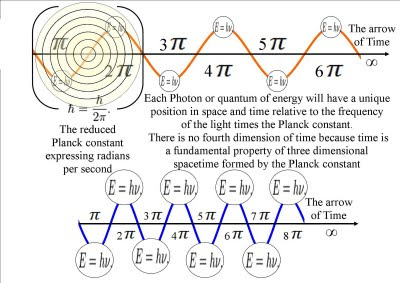Light or EMR forming the arrow of time

~

It is this harmonic oscillation that is at heart of all fractal self similarities. The symmetry and geometry of spacetime will automatically lead to fractals self similarities.Fractal self-similarity in the form of a snowflake.

~

Therefore we see fractal self-similarities at every degree and angle of creation because there is a universal method of pattern formation of organic and non organic matter.Photon electron coupling.

~

Since Newton and Leibniz physics has been based on equations that relate not to the physical object itself but to the rate of change that the object will undergo in a period of time (Differential equations).

These equations on motion and later electromagnetism are very beautiful but there is a small problem and that is we have no understanding of time.

It is normal when people cannot understand something for them to say it does not exist (Atheism). This worked in physics until we looked down into the quantum world of the atoms and found the measurement problem and entanglement.

Only a new theory explaining the arrow of time and the geometry of spacetime can unite Quantum Mechanics and Classical Mechanics giving us one universal law of physics.Each Cantor set is a fractal within infinity.

~

In this theory we can see a World in a Grain of Sand and a Heaven in a Wild Flower, Hold Infinity in the palm of your hand and Eternity in an hour, just as William Blake said.

~
Link to new videos on Quantum Atom Theory on You Tube

Monday, 16 November 2009

Quantum Mechanics the problem of infinities in Quantum Electrodynamics

Video on Quantum Mechanics the problem of infinities in QED

~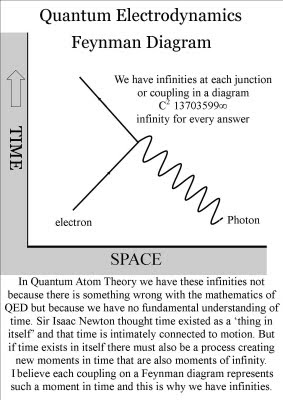The coupling constant 137 cannot be explained and the problem of infinity can only be put right by the odd process of renormalization.
~In Quantum Atom Theory the mathematical infinity is the infinite process of time and the coupling constant 137 can be explained.

~The coupling constant 137 is formed by the continuous process of time creating the broken symmetry of spacetime.

~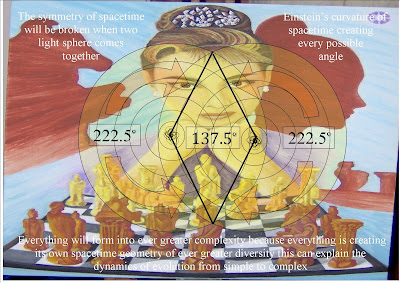The observer will see and feel this as the forward momentum of time and will have free-will within his or her own created spacetime.
~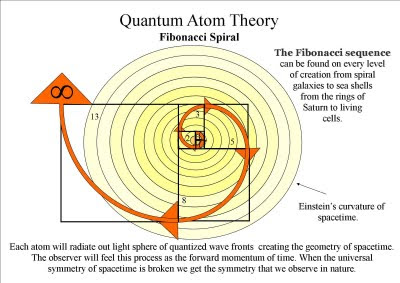We can find the coupling constant 137 by taking any two consecutive numbers of the Fibonacci series and form them into a fraction. Then multiple by 360 to get 222.5 we then subtract this from 360. The result will always be 137 that's why it's a constant. It is the symmetry and geometry of spacetime.

~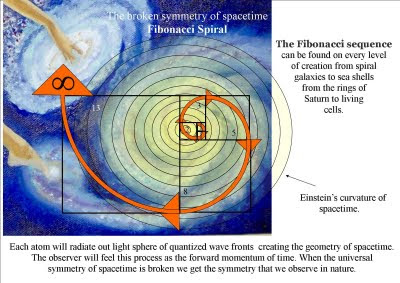But luckily we can also see this in the Beauty of creation within every individual part of our Universe.

~